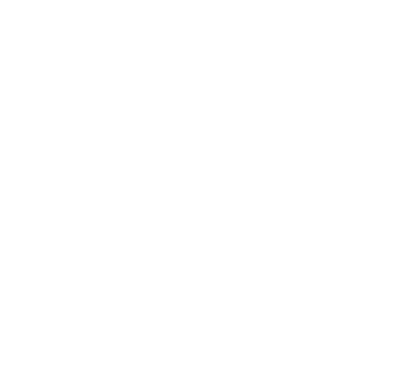Problem 1.

Consider the sequence
\begin{aligned} a_1 & =2^1-3=-1, \ a_2 & =2^2-3=1, \ a_3 & =2^3-3=5, \ a_4 & =2^4-3=13, \ & \vdots \ a_n & =2^n-3, \ & \vdots \end{aligned}
defined for positive integers $n$. Which elements of this sequence are divisible by 5 ? What about 13 ? Are any elements of this sequence divisible by $65=5 \cdot 13$ ? Why or why not?

Proof .

To determine whether an element of the sequence is divisible by $5$, we need to check whether $a_n$ is congruent to $0$ modulo $5$. In other words, we need to check whether $a_n$ leaves a remainder of $0$ when divided by $5$.

We can observe that $2^n$ alternates between leaving a remainder of $2$ and $3$ when divided by $5$. Specifically, $2^n$ leaves a remainder of $2$ when $n$ is even, and a remainder of $3$ when $n$ is odd. Therefore, we have:

\begin{cases}2-3 \equiv-1(\bmod 5) & \text { if } n \text { is even } \ 3-3 \equiv 0(\bmod 5) & \text { if } n \text { is odd }\end{cases}

Problem 2.

To get the echo of a positive integer, we write it twice in a row without a space. For example, the echo of 2023 is 20232023 . Is there a positive integer whose echo is a perfect square? If so, how many such positive integers can you find? If not, explain why not.

Problem 3.

A lattice point is a point $(x, y)$ in the plane, both of whose coordinates are integers. It is easy to see that every lattice point can be surrounded by a small circle which excludes all other lattice points from its interior. It is not much harder to see that it is possible to draw a circle which has exactly two lattice points in its interior, or exactly 3 , or exactly 4, as shown in the picture below.A lattice point is a point $(x, y)$ in the plane, both of whose coordinates are integers.

Do you think that for every positive integer $n$ there is a circle in the plane which contains exactly $n$ lattice points in its interior? Justify your answer.

# 学生速览

PROMYS 是一项为期六周的夏季数学课程，面向从美国和世界各地精心挑选的积极进取的高中学生。PROMYS 成立于 1989 年，是在波士顿大学校园内举办的一项住宿计划，约有 80 名高中生和 25 名本科生辅导员。

#### 关键日期

PROMYS 2023：2023 年7 月 2 日至 8 月 12 日

#### 财政援助和奖学金

PROMYS 认为成本不应成为参与的障碍。请参阅Financial Aid & Scholarships页面以了解全部和部分基于需求的经济援助（包括食宿）、奖学金、助学金和其他奖励。该计划对家庭年收入低于 80,000 美元的国内学生免费。对国际学生的经济援助是根据具体情况考虑的。

#### 合格

• 至少 14 岁
• 已完成 9 年级（或同等学历）
• 尚未注册为全日制学院或大学学生

#### 申请流程

1. 具有挑战性的问题集的解决方案
2. 推荐信
3. 高中成绩单
4. 包含简短答案的申请表

PROMYS 对增加数学和更广泛的科学和技术机会的多样性特别感兴趣。我们强烈鼓励学生申请女性、黑人、拉丁裔/a 或其他在 STEM 中代表性不足的群体。